Multiplication Chart - Definition with Examples

The Complete K-5 Math Learning Program Built for Your Child

• 30 Million Kids

Loved by kids and parent worldwide

• 50,000 Schools

Trusted by teachers across schools

• Comprehensive Curriculum

Aligned to Common Core

What is Multiplication Chart?

Multiplication is repeated addition.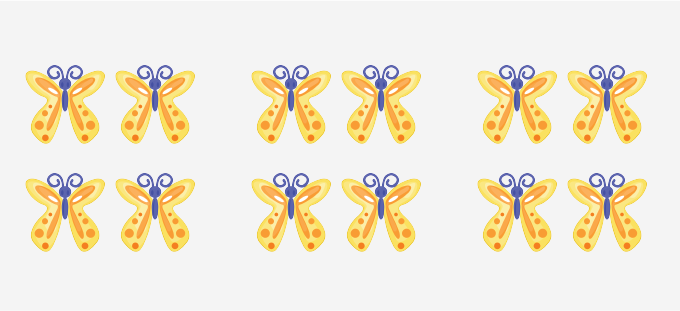There are 3 groups of 4 butterflies each. That is, the total number of butterflies is 3 times 4 or 4 + 4 + 4 or 12.

A simple way to do the day-to-day calculations is through the help of a multiplication chart or times table.

A multiplication chart is a table that shows the products of two numbers. Usually, one set of numbers is written on the left column and another set is written as the top row. The products are listed as a rectangular array of numbers.

For example, a multiplication chart of 10 can be written as: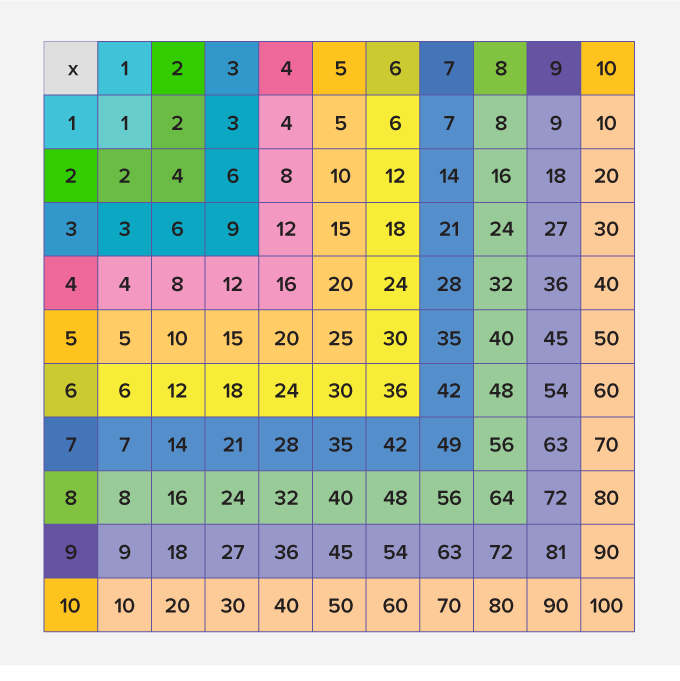.

Reading a multiplication chart

Step 1: Choose the first number from the numbers listed in the left-most column and the second number from the top-most row.

Step 2: Starting from the first number move towards the right and starting from the second number move towards down.  The square where the two numbers meet gives the product!

For example 5 × 4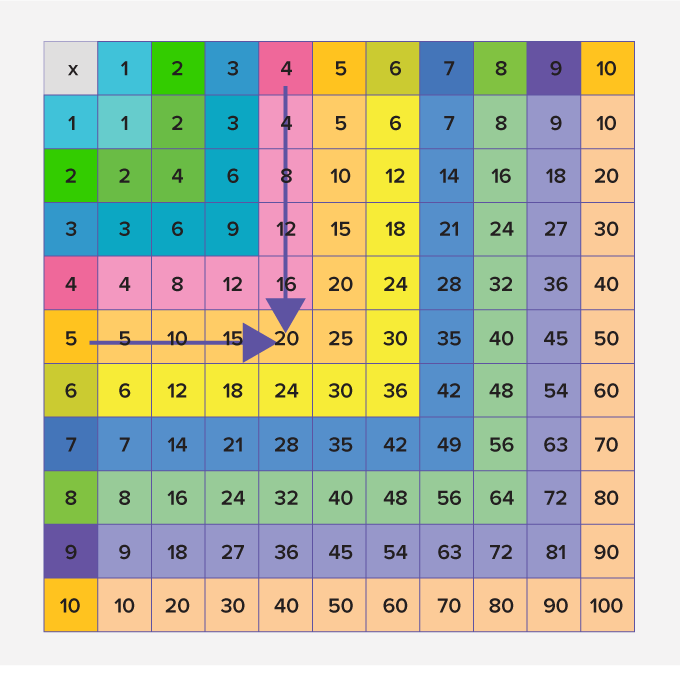Thus, 5 × 4 = 20.

Learning multiplication chart:

A multiplication chart can be divided into two parts, lower times table, and upper times table.

The multiplication tables of 1, 2, 5, and 10 are easier to remember as they follow a pattern. The product of any number with 1 is the number itself whereas the product of any number with 2 is double the number. The ones digits of the multiplication table of 5 alternates between 0 and 5 and it is easy to remember the table of 10 as well because the digit at the ones place is always zero. These parts of the multiplication chart which are easy to remember are called lower times table.

The rest of the table is called the upper times table.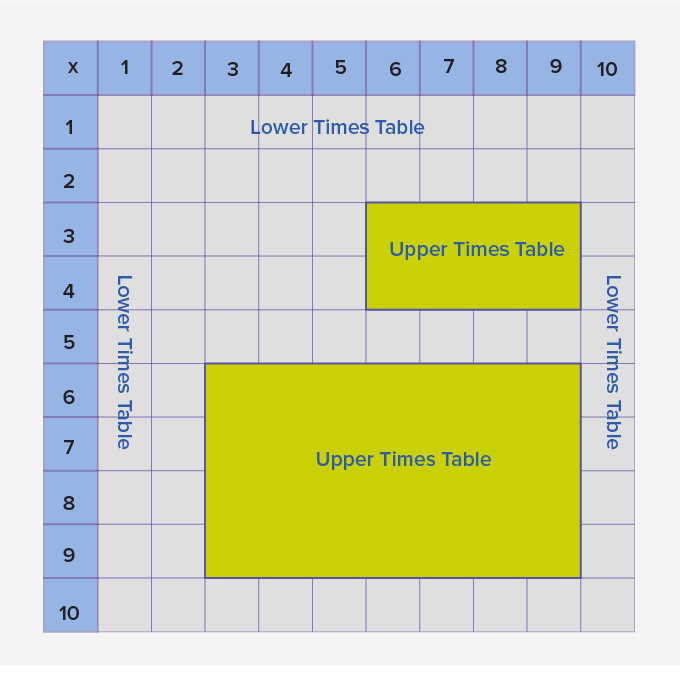The upper times table also can be learned using repeated addition and practice.

One important property of multiplication is that the order in which you multiply any two numbers does not affect the product.

So, in a multiplication chart, for any product, you can find the identical number with the numbers reversed in the statement.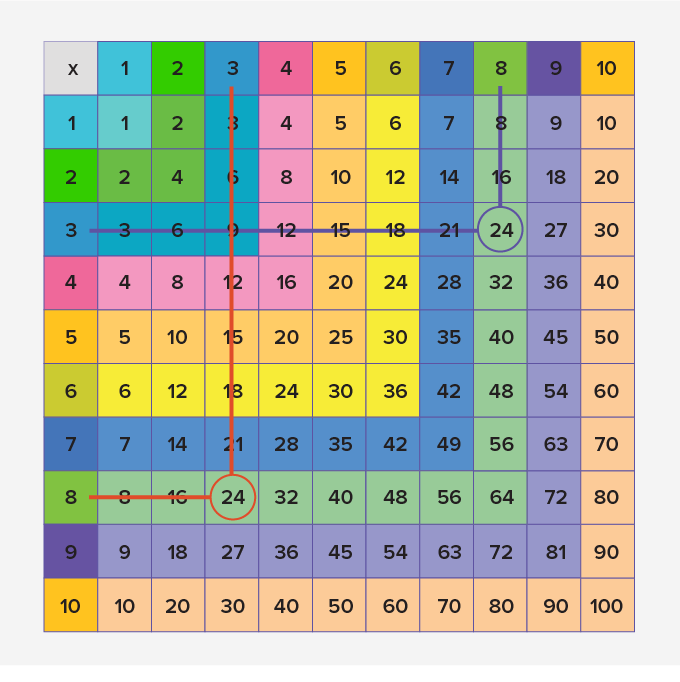Further, you can find many blocks as shown, which are identical but written in the transposed manner.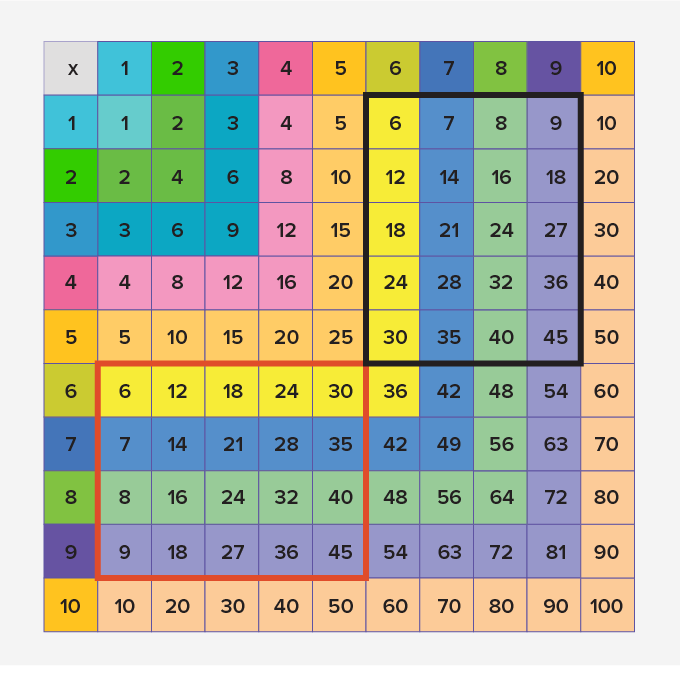Won Numerous Awards & Honors